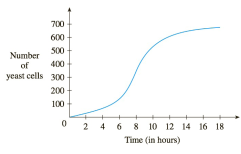Chapter 3.3, Problem 53E

Chapter
Section
Textbook Problem

# A graph of a population of yeast cells in a new laboratory culture as a function of time is shown.(a) Describe how the rate of population increase varies.(b) When is this rate highest?(c) On what intervals is the population function concave upward or downward?(d) Estimate the coordinates of the inflection point.To determine

(a)

To describe:

The rate of population increase varies

Explanation

1) Concept:

Use the graph to observe it and then describe the rate of population increase

2) Formula:

Rate of population increase=Nt

Where

N is the total change in population of yeast cells for the entire time period

t is time in hours

3) Calculation:

A graph of a population of yeast cells in a new laboratory culture as a function of time in hours shows the rate of population of yeast cells with respect to time (t)

To determine

(b)

To find:

The point where the rate of population increase is highest

To determine

(c)

To find:

Intervals on which population function concave upward or downward

To determine

(d)

To find:

The coordinates of inflection point.

### Still sussing out bartleby?

Check out a sample textbook solution.

See a sample solution

#### The Solution to Your Study Problems

Bartleby provides explanations to thousands of textbook problems written by our experts, many with advanced degrees!

Get Started

#### Prove formula (b) of Theorem 3.

Single Variable Calculus: Early Transcendentals

#### 0 1 π It does not exist.

Study Guide for Stewart's Multivariable Calculus, 8th

#### Find if , y = sin 2t.

Study Guide for Stewart's Single Variable Calculus: Early Transcendentals, 8th

#### Given: BEDACDDA Prove: 12

Elementary Geometry for College Students